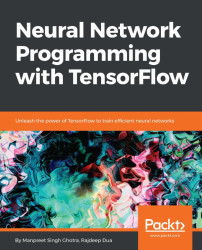•#### Neural Network Programming with Tensorflow#### Overview of this book

If you're aware of the buzz surrounding the terms such as "machine learning," "artificial intelligence," or "deep learning," you might know what neural networks are. Ever wondered how they help in solving complex computational problem efficiently, or how to train efficient neural networks? This book will teach you just that. You will start by getting a quick overview of the popular TensorFlow library and how it is used to train different neural networks. You will get a thorough understanding of the fundamentals and basic math for neural networks and why TensorFlow is a popular choice Then, you will proceed to implement a simple feed forward neural network. Next you will master optimization techniques and algorithms for neural networks using TensorFlow. Further, you will learn to implement some more complex types of neural networks such as convolutional neural networks, recurrent neural networks, and Deep Belief Networks. In the course of the book, you will be working on real-world datasets to get a hands-on understanding of neural network programming. You will also get to train generative models and will learn the applications of autoencoders. By the end of this book, you will have a fair understanding of how you can leverage the power of TensorFlow to train neural networks of varying complexities, without any hassle. While you are learning about various neural network implementations you will learn the underlying mathematics and linear algebra and how they map to the appropriate TensorFlow constructs.
Title PageCreditswww.PacktPub.comCustomer FeedbackPrefaceFree Chapter
Maths for Neural NetworksDeep Feedforward NetworksOptimization for Neural NetworksConvolutional Neural NetworksRecurrent Neural NetworksGenerative ModelsDeep Belief NetworkingAutoencodersResearch in Neural NetworksGetting started with TensorFlow## Autoencoder algorithms

In the following notation, `x` is the input, `y` is the encoded data, `z` is the decoded data, `σ` is a nonlinear activation function (sigmoid or hyperbolic tangent, usually), and `f(x;θ)` means a function of `x` parameterized by `θ`.

The model can be summarized in the following way:

The input data is mapped to the hidden layer (encoding). The mapping is usually an affine (allowing for or preserving parallel relationships.) transformation followed by a non-linearity:

`y = f(x;θ) = σ(Wx+b)y = f(x;θ) =σ(Wx+b)`

The hidden layer is mapped to the output layer, which is also called decoding. The mapping is an affine transformation (affine transformation is a linear mapping method that preserves points, straight lines, and planes) optionally followed by a non linearity. The following equation explains this:

`z = g(y;θ′) = g(f(x;θ);θ′) = σ(W′y+b′)`

In order to reduce the size of the model, tied weights can be used, which means that the decoder weights matrix is constrained and can be the transpose...